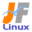Linux Kernel 2.6 Documentation: /usr/src/linux/Documentation/BUG-HUNTING

BUG-HUNTING

Kernel\$B\$N(BBug\$B\$r8+\$D\$1\$kJ}K!(B [\$B%W%l%\$%s%F%-%9%HHG(B]
• \$B86Cx:n
• \$BK]Lu
• \$B%P!<%8%g%s(B: 2.6.5
• \$BK]LuF|;~(B: 2004/03/23
```

[Sat Mar  2 10:32:33 PST 1996 KERNEL_BUG-HOWTO lm@sgi.com (Larry McVoy)]

This is how to track down a bug if you know nothing about kernel hacking.
It's a brute force approach but it works pretty well.

\$B\$3\$NJ8=q\$G\$O!"\$"\$J\$?\$,%+!<%M%k\$N%O%C%-%s%0\$K\$D\$\$\$FCN\$i\$J\$\$>l9g\$K(B
\$B\$I\$N\$h\$&\$K\$7\$F%P%0\$r8+\$D\$1\$@\$9\$+!"\$H\$\$\$&;v\$K\$D\$\$\$F@bL@\$7\$F\$\$\$^\$9!#(B
\$BNO6H\$G\$O\$"\$j\$^\$9\$,!\$\$A\$c\$s\$H;H\$(\$kJ}K!\$G\$9!%(B

You need:

\$BI,MW\$J\$N\$O!'(B

. A reproducible bug - it has to happen predictably (sorry)
. All the kernel tar files from a revision that worked to the
revision that doesn't

\$B!&:F8=\$9\$k%P%0(B \$B!](B \$B:F8=\$5\$;\$kJ}K!\$,\$o\$+\$C\$F\$\$\$kI,MW\$,\$"\$j\$^\$9!#(B
\$B!&@5>oF0:n\$9\$k\$b\$N\$+\$iF0:n\$7\$J\$\$\$b\$N\$^\$G\$NA4%j%S%8%g%s\$N(B
\$B%+!<%M%k(B tar \$B%U%!%\$%k(B

You will then do:

\$B\$=\$l\$+\$io\$KF0:n\$9\$k\$@\$m\$&\$H;W\$o\$l\$k%j%S%8%g%s\$r%j%S%k%I!"(B
\$B%\$%s%9%H!<%k\$7\$FF0:n\$r8!>Z\$7\$F\$/\$@\$5\$\$!#(B
\$B!&%+!<%M%kFb\$r%P%\$%J%j!<%5!<%A!JLuCm!'%P%\$%J%j!<%U%!%\$%k\$r%5!<%A(B
\$B!!\$9\$k\$3\$H\$G\$O\$J\$/!"L\E*\$N?tCM!":GBg!?:G>.CM\$NHf3S\$r9T\$\$!"Cf4VCM\$r(B
\$B!!.CM\$rHf3S!&!&!&\$H7+\$jJV\$7\$F%5!<(B
\$B!!%A\$r9T\$&\$3\$H\$G\$9!##2J,C5:w\$H\$b8@\$o\$l\$^\$9!K\$7\$F\$I\$N%j%S%8%g%s(B
\$B!!\$+\$i%P%0\$,4^\$^\$l\$F\$\$\$k\$+\$r8+\$D\$1\$F\$/\$@\$5\$\$!#Nc\$(\$P!"(B1.3.28 \$B\$K\$O(B
\$B!!%P%0\$,L5\$\$\$,!"(B1.3.69 \$B\$K\$O%P%0\$,\$"\$k\$3\$H\$,\$o\$+\$C\$?\$H\$7\$^\$9!#(B
\$B!!oF0:n\$7\$?>l9g!"(B.50\$B!A(B.69\$B\$NCf4V\$N!"F0:n\$7\$J\$+\$C\$?>l9g(B
.28\$B!A(B.50 \$B\$NCf4V\$N%j%S%8%g%s\$GF1\$8\$h\$&\$K7+\$jJV\$7\$^\$9!#\$3\$&\$9\$k(B
\$B\$3\$H\$K\$h\$C\$F!"%P%0\$,:.F~\$5\$l\$?%j%S%8%g%s\$r8+\$D\$1\$k\$3\$H\$,\$G\$-\$^(B
\$B\$9!#>e5-\$h\$jNI\$\$J}K!\$G3:Ev%j%S%8%g%s\$r8+\$D\$1\$k;v\$b\$G\$-\$^\$9\$,!"(B
\$B\$=\$N>l9g\$O>/!9%H%j%C%-!<\$JJ}K!\$K\$J\$k\$G\$7\$g\$&!#(B

. Narrow it down to a subdirectory

\$B!&JQ99E@\$r%5%V%G%#%l%/%H%jC10L\$K9J\$j9~\$`(B

- Copy kernel that works into "test".  Let's say that 3.62 works,
but 3.63 doesn't.  So you diff -r those two kernels and come
up with a list of directories that changed.  For each of those
directories:

- \$B@5>o\$KF0:n\$9\$k%+!<%M%k\$r(B "test" \$B%G%#%l%/%H%j\$K%3%T!<\$7\$^\$9!#(B
\$BNc\$(\$P(B 3.62 \$B\$,@5>oF0:n\$9\$k\$b\$N\$G!"(B3.63 \$B\$,F0:n\$7\$J\$\$\$b\$N\$@\$H\$7\$^(B
\$B\$9!#(B2 \$B\$D\$N%+!<%M%k\$r(B diff -r \$B\$7\$F!"JQ99\$5\$l\$?%G%#%l%/%H%j\$N(B
\$B%j%9%H\$rF@\$^\$9!#\$=\$N%j%9%HFb\$N3F%G%#%l%/%H%j\$KBP\$7\$F!"(B

Copy the non-working directory next to the working directory
as "dir.63".
One directory at time, try moving the working directory to
"dir.62" and mv dir.63 dir"time, try

\$B@5>oF0:n\$7\$J\$\$%G%#%l%/%H%j\$r@5>oF0:n\$9\$k%G%#%l%/%H%j\$H(B
\$BF1\$83,AX\$K(B "dir.63" \$B\$J\$I\$H\$\$\$&L>A0\$K\$7\$F%3%T!<\$7\$^\$9!#(B
\$B@5>o\$KF0:n\$9\$k%G%#%l%/%H%j\$H!"F0:n\$7\$J\$\$%G%#%l%/%H%j\$N(B
\$BL>A0\$rDj\$9\$k\$H!"JQ99\$5\$l\$?E@\$r%G%#%l%/%H%j\$NCf\$@\$1\$K\$9\$k\$3(B
\$B\$H\$,\$G\$-\$k\$O\$:\$G\$9!#(B

Problems: changes in header files may have occurred; I've
found in my case that they were self explanatory - you may
or may not want to give up when that happens.

\$BLdBjE@!'%X%C%@!<%U%!%\$%kFb\$,JQ99\$,\$5\$l\$?2DG=@-\$b\$"\$j\$^\$9!#(B
\$B;d\$N\$_\$D\$1\$?%1!<%9\$N>l9g\$O@bL@\$NI,MW\$b\$J\$\$\$/\$i\$\$\$O\$C\$-\$j(B
\$B\$7\$?\$b\$N\$G\$7\$?\$,(B - \$BD|\$a\$?\$/\$J\$k%1!<%9\$b\$"\$k\$+\$b\$7\$l\$^\$;\$s!#(B

. Narrow it down to a file

\$B!&JQ99E@\$r%U%!%\$%kC10L\$K9J\$j9~\$`(B

- You can apply the same technique to each file in the directory,
hoping that the changes in that file are self contained.

- \$B%G%#%l%/%H%jFb\$N3F%U%!%\$%k\$KBP\$7\$F!"JQ99E@\$O\$=\$N%U%!%\$%kFb\$K(B
\$B4^\$^\$l\$F\$\$\$k\$H2>Dj\$7\$^\$9(B

. Narrow it down to a routine

\$B!&JQ99E@\$r=hM}C10L\$GC5\$9(B

- You can take the old file and the new file and manually create
a merged file that has

- \$B?75l\$N%j%S%8%g%s\$N%U%!%\$%k\$+\$i%k!<%A%s\$rpJs(B \$B!](B \$B%+!<%M%k\$N%j%S%8%g%s!"%P%0\$N@bL@!"JQ99E@\$NC5:w\$r9T\$\$(B
\$B8+\$D\$1\$@\$7\$?5!G=3HD%\$NItJ,(B \$B!](B \$B\$r\$=\$N!J%P%0\$N=P\$?!K%;%/%7%g%s\$N%a%s%F%J!<(B
\$B\$@\$H;W\$o\$l\$k?M\$KEO\$7\$F\$/\$@\$5\$\$!#(Blinux.dev.kernel \$B\$K%]%9%H\$7\$F\$b\$h\$\$\$G\$7\$g\$&!#(B
\$B4{\$KLdBjE@\$NHO0O\$r9J\$C\$F\$5\$(\$\$\$l\$P\$G\$9\$,!#(B

If you get it down to a routine, you'll probably get a fix in 24 hours.

\$B\$b\$7\$bLdBjE@\$NHO0O\$r%k!<%A%s\$K\$^\$G9J\$C\$F\$\$\$k\$N\$G\$"\$l\$P!"\$*\$=\$i\$/(B 24 \$B;~4V(B
\$B0JFb\$KLdBjE@\$O=\$I|\$5\$l\$k\$G\$7\$g\$&!#(B

My apologies to Linus and the other kernel hackers for describing this
brute force approach, it's hardly what a kernel hacker would do.  However,
it does work and it lets non-hackers help fix bugs.  And it is cool
because Linux snapshots will let you do this - something that you can't
do with vendor supplied releases.

Linux \$B\$dB>\$N%+!<%M%k%O%C%+!

Linux \$B%+!<%M%k(B 2.6 \$BIUB0J8=q0lMw\$XLa\$k(B

JF Project \$B%a%\$%s%Z!<%8\$X(B
JF Project \$BA4HL\$K4X\$o\$k\$40U8+\$4MWK>\$O(B jf@listserv.linux.or.jp \$B\$^\$G\$*4j\$\$\$7\$^\$9(B.

```